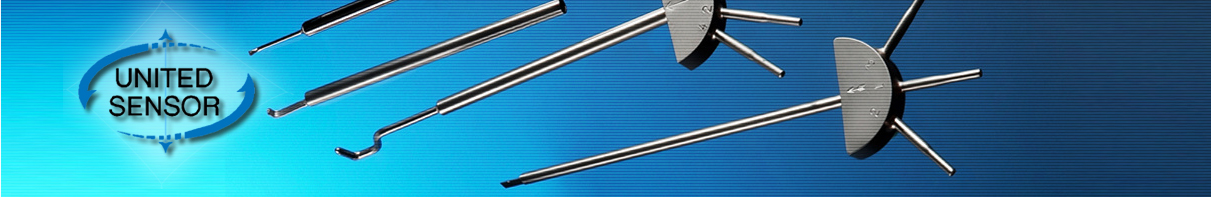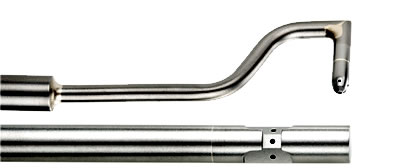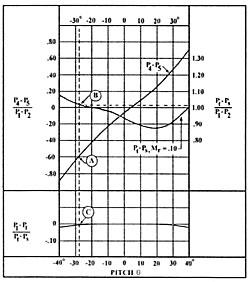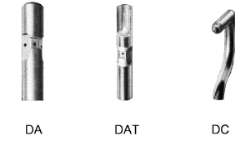# 3 Dimension Directional Probes

본문 바로가기
사이트 내 전체검색3 Dimension Directional Probes

# 3 Dimension Directional Probes

## 3-Dimensional ProbesUnited Sensor 3 Dimensional Directional probes measure yaw and pitch angles of fluid flow, as well as total and static pressures and total temperature.Fig 1. Typical manometer connections for DA or DC probes.

Each probe has five measuring holes located on its tip. A centrally located pressure hole measures pressure P1, while two lateral pressure holes measure pressures P2 and P3. If the probe is rotated by a Traverse Unit (Fig. 1) until P2 = P3 as indicated on a manometer or other sensitive pressure indicator, the yaw angle of flow is then indicated by the traverse unit scale (see Manual Traverse Unit ).Fig 2. Typical calibration of DA probe versus pitch angle.

When the yaw angle has been determined an additional differential pressure P4 - P5 is measured by pressure holes located above and below the total pressure (P1) hole. Pitch angle is determined by calculating (P4 - P5)/ (P1 - P2) and using the calibration curve for the individual probe (e.g. Curve A, Figs. 2 and 3). At any particular pitch angle the velocity pressure coefficient (Pt - Ps)/ (P1 - P2) and total pressure coefficient (P1 - Pt)/ (Pt - Ps) can be read from curves B and C, while Pt - Ps and Ps can be calculated. In the case of Type DAT probes, a half shielded thermocouple sensor at the probe tip provides total temperature measurements at the same point in the stream as fluid pressure and flow direction measurements.Fig 3. Typical calibration of DC probe versus pitch angle.• 게시물이 없습니다.

## 접속자집계

오늘
54
어제
65
최대
1,509
전체
137,668경기도 하남시 조정대로 150(덕풍동 762번지) 아이테코 오렌지존 1024호 | 전화 : 031) 790-4090 - 1 | 팩스 : 031) 790-4093 | E-mail : jjsys@jungjinsystem.com
Copyright © JUNGJIN. All rights reserved.